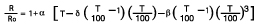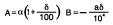### Temperature Coefficient (Alpha)

Temperature coefficient, or Alpha, is the term given to the average resistance/temperature relationship of an RTD over the temperature span of 0-100°C and is expressed as ohm/ohm/0°C. The formula for determining Alpha is:

 A = R(100°C) – R(0°C) 100R (0°C)

Typical Temperature Coefficients are:

 .003926 (99.999% Pure Plat. – Laboratory Grade) .00320 (MIL-T-24388) .00391 .003915 .003902 Sometimes referred to as American standards, although no standards exist. .003850 Din Standard 43760-widely used in industrial applications. B.S. 1904:1964

Note: SAMA Standard RC21-4-1966, has a temperature coefficient of .003923. The SAMA 100 ohm (Nominal) element only has a resistance of 98.129 ohms at 0°C. This element is in common use and should not be confused with the more commonly used elements having a 100 ohm resistance at 0°C.

 Nickel Copper Nickel/Iron .00672 .00427 (Balco) .00618 .00519

Platinum is by far the most commonly used material in RTD probes. The other materials are used where the higher resistance change or the non-linearity of their curves are advantageous.

It becomes obvious that since Alpha is a value developed using the variables of R(0°C) & R(100°C) that the Alphas noted above are not absolutes.They do however commonly serve as adequate specifications for the standard elements.

Alpha serves as an integral component in developing resistance versus temperature tables. The R/T table for platinum sensors published at the back of this catalog were developed using the Callendar-Van Dusen equation which corrects for the departure from linearity at temperatures other than 0-100°C which is stated by Alpha.

Callendar-Van Dusen EquationWhere:
T = Temperature (°C)
R = Resistance at temperature T
R0 = Resistance at 0°C

α = Constant (see formula above)
δ = Constant (typical value 1.5)
β = Constant (typical value 0.11 for temperatures less than 0°C; value is zero for temperatures over 0°C)
a useful form of this equation to calculate the resistance at a given temperature at and above 0°C is:

RT = RO (1 + AT + BT2)RT = Resistance at temperature T
RO, T, a and d are defined in the first equation.

### Accuracy – Interchangeability

The terms accuracy and interchangeability are used jointly when considering the accuracy of an RTD. The factors affecting the accuracy of an RTD measurement excluding the accuracy of the readout instrument, is the relationship of the “as built” sensor to the resistance vs. temperature curve when compared to the assumed curve (the resistance vs. temperature table), and any aging or other environment effects on the sensor.

It is impossible to manufacture on a production basis sensors that will adhere to the calculated value of their resistance vs. temperature tables. There are three terms to be considered to understand why. They are:

Proportional Error –
The error caused by the deviation of the sensor’s actual temperature coefficient (Alpha) from the reference value. This error exists because of normal manufacturing tolerances in the alloying of the sensor material.

Adjustment Error –
The error caused by deviations of resistance at 0°C from the reference values. For example – a standard tolerance at 0°C for a 100 ohm platinum element is ± 0.12%. a wider tolerance of ± 0.5% and tighter tolerance of ± 0.06% and ± 0.03% are available but are somewhat less expensive and appreciably higher in cost respectively.

Intrinsic Error –
Simply the sums of the proportional and adjustment errors. The intrinsic error of an element will influence its relationship to the published resistance/temperature table. Deviations are a function of temperature and accuracy statements cannot be given that will cover the entire useful range of a sensor. Therefore, an “interchangeability factor” is stated for cardinal temperatures throughout the range. The interchangeability tolerance for a 100 ohm platinum element (± 0.12%) is shown in the table below.

 Temp Tolerance C ± 0° C ± OHMS -.200 1.3 0.56 -100 0.8 0.32 0 0.3 0.12 100 0.8 0.30 200 1.3 0.48 300 1.8 0.64 400 2.3 0.79 500 2.8 0.93 600 3.3 1.06 700 3.8 1.17 100 ohm ± .12% @ 0°C Element

Note: Not all sensors are usable at all the temperatures shown. The tolerances are applicable up to the maximum temperature of a given sensor.